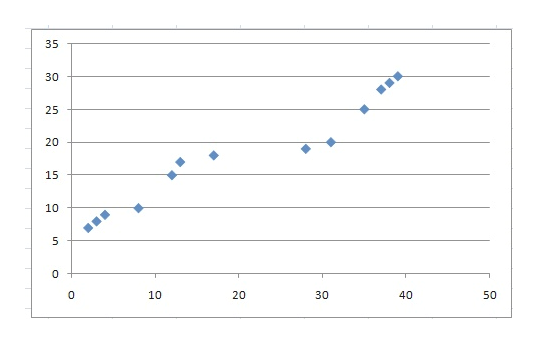# What does it mean when two variables are described as “positively correlated”?

Question
21 views

What does it mean when two variables are described as “positively correlated”?

check_circle

Step 1

In statistical analysis to measure the relation between two bivariate data, then if the change of a variable affects a change in the other variable, then this relationship is called correlation.

If the two variable deviate in the same direction, that is if the increase ( or decrease) in one results in a corresponding increase (or decrease) in the other , correlation is said to be positive.

If the two variable deviate in the opposite direction, that is if the decrease ( or increase) in one results in a corresponding increase (or decrease) in the other , correlation is said to be negative.

In order to draw the positive correlation we can use a scatter diagram as follows:help_outlineImage Transcriptionclose35 30 25 20 15 10 5 0 0 10 30 40 50 20 LO fullscreen
Step 2

In mathematically we can writ...

### Want to see the full answer?

See Solution

#### Want to see this answer and more?

Solutions are written by subject experts who are available 24/7. Questions are typically answered within 1 hour.*

See Solution
*Response times may vary by subject and question.
Tagged in

### Measures